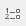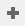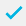# Increasing or decreasing the size of a sample group

You could change the size of the sample group in the same way that you changed the proportions (balance) of a sample group. You would only need to choose different target figures (e.g. set the target number of males to be 150 and set the target number of females to be 100).

If you simply want to increase or decrease the number of cases in the survey, you could follow the steps below, which divides the target number by the actual number. In the example below, 384 is the actual number of respondents and 500 is the target number:

1. Clickto display the Variables window.
2. Clickto add a new variable.
3. Specify the Variable Details:
• Name: V8
• Label: Inflated cases
• Type: Derived (the variable will derive its data from other existing variables).
• Response: Quantity (the response will be a numeric value for each case).
• Decimals: To access the Decimals field, click on. Change the figure from 0 to 3.
4. Specify the Code Details to calculate the higher base. As with the creation of a weight, the calculation is going to divide the target figure by the actual figure.
1. Clickto save the variable.
2. Clickto display the Analysis Definition dialog to create a table.
3. Specify the Analysis as Q2, which is the variable to be weighted.
4. Specify the Weight as V8.
1. Click OK and the weighted table will be built.

The inclusion of the weight will multiply the number of respondents in each response code by the value in V8.

You may also need to set the Decimal Places of Calculations to 3 to avoid rounding errors. Select the Cells tab on the Analysis Definition dialog and alter the Calculations d.p. number, if required.

Contents